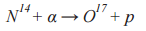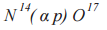Formats of nuclear reactions recording

The recording format of nuclear reactions could be different, depending on the symbols used. For example, often there are the following formats of recording:

H11 (protium; proton) – p

H21 (deuterium; deuton, deuteron) – d (D)

H31 (tritium; triton) ‒ t (T)

H42 (Helium-4, α-particle) ‒ α

It is also worth noting that the atomic number of the element can be omitted when recording the nuclear reaction, and only a total mass number is indicated, pointing to the isotope. For example, the above mentioned formula can be written as follows:In addition, the following abbreviated recording of formula of nuclear reaction is adopted in nuclear chemistry: A (a, b) B, where A - is the initial nucleus; B –the nucleus that is produced as a result of reaction; a is a particle which interacted with the nucleus; b - is a particle, produced as a result of interaction (thrown out from the nucleus). The above formula in this case would be as follows:We will further (see tables below) consider several types of nuclear reactions and calculate the number of phantom Po particles. There is a full recording of the reaction and the calculation of phantom Po particles in the second column of the table. There is an example of the reaction the way it is recorded in the literature in the third column. The literary sources are below the table indicating the page where the reaction formula is.